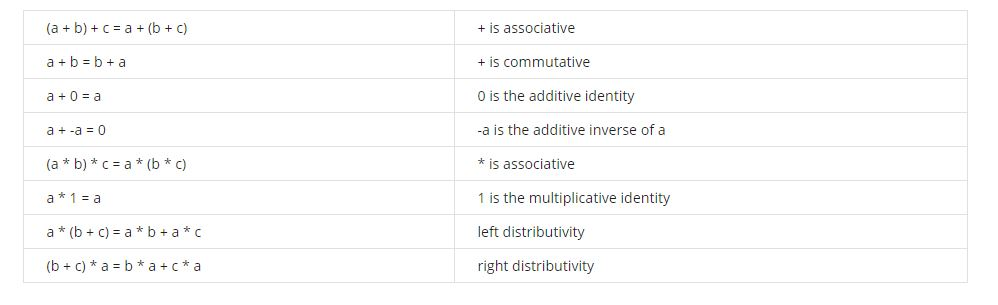## Motivating Example & Type-Directed Programming

• 类型为 T
• 有implicit关键字
• 函数调用时可见, 或者在和T相关的伴生类中有定义

• 具体类型大于泛型
• 子类大于父类

Implicit Query

## Type Classes

type class 可以帮助我们实现多态

• 自反 compare(x, y) 和 compare(y, x)的符号应该相反
• 传递 如果 compare(x, y) < 0, compare(y, z) < 0 那么 compare(x, z) < 0
• 一致 如果 x, y 相等， 那么 compare(x, z) 与 compare(y, z) 的符号应该相同

In mathematics, a ring is one of the fundamental algebraic structures used in abstract algebra. It consists of a set equipped with two binary operations that generalize the arithmetic operations of addition and multiplication. Through this generalization, theorems from arithmetic are extended to non-numerical objects such as polynomials, series, matrices and functions.

This structure is so common that, by abstracting over the ring structure, developers could write programs that could then be applied to various domains

(arithmetic, polynomials, series, matrices and functions).

<R, +, *>满足以下定律

• 是一个加法上的交换群
• 是一个乘法上的Monid
• 满足乘法上的分配律

$$\forall a, b ∈ G, 有a · b \in G$$

$$\forall a, b, c ∈ G, 有 (a · b) · c = a · (b · c)$$

$$\exists e ∈ G， 使得 \forall a∈G，都有 e · a = a · e = a$$

$$\forall a \in G, \exists b \in G, 使得 a · b = b · a = e$$

$$\forall a, b \in G, a · b = b · a$$

Monid需要有如下性质

• 有单位元
• 满足结合律

• 左分配律 a ⋅ (b + c) = (a · b) + (a · c)
• 右分配律 (b + c) · a = (b · a) + (c · a)## Implicit Conversions

14.seconds 会被编译成new HasSeconds(15).seconds

• T不符合表达式的预期类型
• 在选择e.m中，如果成员m在T上不可访问
• 在选择e.m（args）中，如果成员m在T上可访问，但不适用于参数args

## 编程作业： Json编码解码器

### 问题背景

Json的数据结构定义如下

Encoder做的工作，就是把不同类型的数据转换成Json class的类型

### Our Tasks

• Encoder[String], Encoder[Boolean]
• Decoder[Int], Decoder[String], Decoder[Boolean]

• Decoder[Person]
• Encoder[Contacts], Decoder[Contacts]

### 附加题

• 你能显示的写出编译器推断Encoder[List[Person]]的结果吗

• 可以对Option的字段定义编码/解码器吗

由下一个问题，知可以

• 可不可以对类型A定义一个隐式实例Encoder[Option[A]]（就像Encoder[List[A]]一样）

蹩脚地模仿着实现